## Excel POISSON Function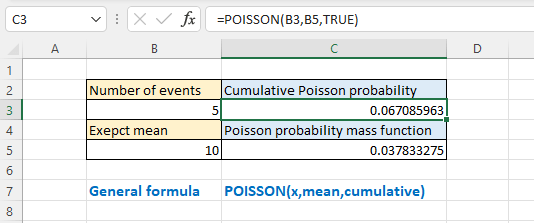### Description

The Excel POISSON function returns the Poisson distribution which is used to predict the number of events occurring over a specific time, e.g., the number of people arriving at the market in 10 minutes.

Note: This function has been replaced with POISSON.DIST function that may provide improved accuracy after Excel 2010 version. This function is still can be available but considering it may be not available in the future, you should better study to use the new function from now.

Formula syntax

POISSON(x,mean,cumulative)

Arguments

 X: Required, the number of events. Mean: Required, the expected numeric value. Cumulative: Required, a logical value that determines the form of the probability distribution returned. TRUE: returns the cumulative Poisson probability that the number of random events occurring will be between 0 and x inclusive; FALSE: returns the Poisson probability mass function that the number of events occurring will be exactly x.

### Returns

POISSON function returns a numeric value.

### Remarks

1. If x is not an integer, it will be truncated.

2. If x or mean is non-numeric, the #VALUE! error value will be returned.

3. If x < 0 or mean < 0, the #NUM! error value will be returned.

4. POISSON.DIST is calculated as the following equations:

For cumulative = TRUE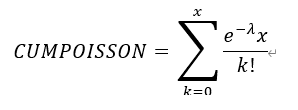for cumulative = FALSE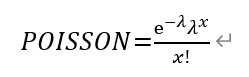Excel 2007

### Usage and Examples

Basic usage

Based on the given number of events and expected mean in cells B3 and B5, you can get the cumulative Poisson probability (cumulative as TRUE) and Poisson probability mass function (cumulative as FALSE) by using the POISSON function as below:

=POISSON (B3,B5,TRUE)

Or directly use numbers in the function

=POISSON (5,10,TRUE)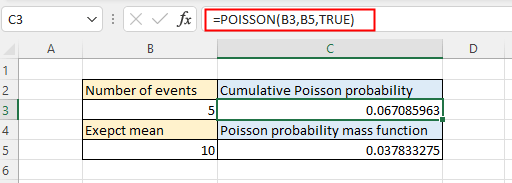=POISSON (B3,B5,FALSE)

Or directly use numbers in the function

=POISSON (5,10,FALSE)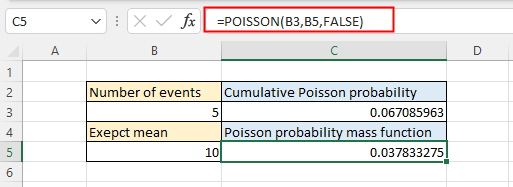Press Enter key to get result.

#### Other Functions:

• Excel F.INV Function
The Excel F.INV function returns the inverse of F probability distribution. If p (probability) = F.DIST(x,…), then F.INV(p,…)= x.

• Excel FDIST Function
The Excel FDIST Function returns the (right-tailed) F probability distribution which is usually used to measure the degree of diversity between two data sets.

• Excel EXPON.DIST Function
EXPON.DIST function used in Excel 2010, returns the exponential distribution based on the given x value and the parameter of the distribution.

• Excel F.DIST Function
The Excel F.DIST function returns the F probability distribution which is usually used to measure the degree of diversity between two data sets.

### The Best Office Productivity Tools

#### Kutools for Excel - Helps You To Stand Out From Crowd

 Popular Features: Find, Highlight or Identify Duplicates  |  Delete Blank Rows  |  Combine Columns or Cells without Losing Data  |  Round without Formula ... Super VLookup: Multiple Criteria  |  Multiple Value  |  Across Multi-Sheets  |  Fuzzy Lookup... Adv. Drop-down List: Easy Drop Down List  |  Dependent Drop Down List  |  Multi-select Drop Down List... Column Manager: Add a Specific Number of Columns  |  Move Columns  |  Toggle Visibility Status of Hidden Columns  |  Compare Columns to Select Same & Different Cells ... Featured Features: Grid Focus  |  Design View  |  Big Formula Bar  |  Workbook & Sheet Manager | Resource Library (Auto Text)  |  Date Picker  |  Combine Worksheets  |  Encrypt/Decrypt Cells  |  Send Emails by List  |  Super Filter  |  Special Filter (filter bold/italic/strikethrough...) ... Top 15 Toolsets:  12 Text Tools (Add Text, Remove Characters ...)  |  50+ Chart Types (Gantt Chart ...)  |  40+ Practical Formulas (Calculate age based on birthday ...)  |  19 Insertion Tools (Insert QR Code, Insert Picture from Path ...)  |  12 Conversion Tools (Numbers to Words, Currency Conversion ...)  |  7 Merge & Split Tools (Advanced Combine Rows, Split Excel Cells ...)  |  ... and more

Kutools for Excel Boasts Over 300 Features, Ensuring That What You Need is Just A Click Away...#### Office Tab - Enable Tabbed Reading and Editing in Microsoft Office (include Excel)

• One second to switch between dozens of open documents!
• Reduce hundreds of mouse clicks for you every day, say goodbye to mouse hand.
• Increases your productivity by 50% when viewing and editing multiple documents.
• Brings Efficient Tabs to Office (include Excel), Just Like Chrome, Edge and Firefox.